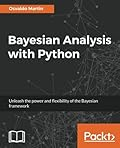# Bayesian Analysis with PythonBayesian Analysis with Python
By 作者: Osvaldo Martin
ISBN-10 书号: 1785883801
ISBN-13 书号: 9781785883804
Release Finelybook 出版日期: 2016-11-25
pages 页数: 368

Book Description to Finelybook sorting

Learn
Understand the essentials Bayesian concepts from a practical point of view
Learn how to build probabilistic models using the Python library PyMC3
Acquire the skills to sanity-check your models and modify them if necessary
Add structure to your models and get the advantages of hierarchical models
Find out how different models can be used to answer different data analysis questions
When in doubt, learn to choose between alternative models.
Predict continuous target outcomes using regression analysis or assign classes using logistic and softmax regression.
Learn how to think probabilistically and unleash the power and flexibility of the Bayesian framework
The purpose of this book is to teach the main concepts of Bayesian data analysis. We will learn how to effectively use PyMC3, a Python library for probabilistic programming, to perform Bayesian parameter estimation, to check models and validate them. This book begins presenting the key concepts of the Bayesian framework and the main advantages of this approach from a practical point of view. Moving on, we will explore the power and flexibility of generalized linear models and how to adapt them to a wide array of problems, including regression and classification. We will also look into mixture models and clustering data, and we will finish with advanced topics like non-parametrics models and Gaussian processes. With the help of Python and PyMC3 you will learn to implement, check and expand Bayesian models to solve data analysis problems.

Features
Simplify the Bayes process for solving complex statistical problems using Python;
Tutorial guide that will take the you through the journey of Bayesian analysis with the help of sample problems and practice exercises;
Learn how and when to use Bayesian analysis in your applications with this guide.

contents
1 Thinking Probabilistically – A Bayesian Inference Primer
2 Programming Probabilistically – A PyMC3 Primer
3 Juggling with Multi-Parametric and Hierarchical Models
4 Understanding and Predicting Data with Linear Regression Models
5 Classifying Outcomes with Logistic Regression
6 Model Comparison
7 Mixture Models
8 Gaussian Processes

ZIP压缩文内包含(PDF+EPUB+AZW3+MOBI+Code)其一

### 评论 2

1. #1

Bayesian Analysis with Python
By 作者: Osvaldo Martin
ISBN-10 书号: 1785883801
ISBN-13 书号: 9781785883804 这本无法下载，可以更新一下吗？谢谢！

jameshen 3周前 (08-30)回复
• @jameshen 已更新

admin 3周前 (08-30)回复

### 觉得文章有用就打赏一下文章作者

#### 支付宝扫一扫打赏#### 微信扫一扫打赏• QQ咨询
• 回顶